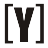# Inches to cm converter

Centimeters result: cm
Meters+centimeters result:
 m
cm
Calculation:

Example:

Inches (Fraction) to cm:

Convert 1/8 inches to cm?
Calculation: 0.125″ × 2.54 = 0.3175 cm
1/8 inches = 0.3175 cm

Convert 1/2 inches to cm?
Calculation: 0.5″ × 2.54 = 1.27 cm
1/2 inches = 1.27 cm

Convert 1/4 inches to cm?
Calculation: 0.25″ × 2.54 = 0.635 cm
1/4 inches = 0.635 cm

Convert 1 3/4 inch to cm?
Calculation: 1.75″ × 2.54 = 4.445 cm
1 3/4 inches = 4.445 cm

Convert 2 5/8 inch to cm?
Calculation: 2.625″ × 2.54 = 6.6675 cm
2 5/8 inches = 6.6675 cm

Convert 3 3/2 inch to cm?
Calculation: 4.5″ × 2.54 = 11.43 cm
3 3/2 inches = 11.43 cmYoosFuhl.com Would you like to receive notifications on latest updates? No Yes